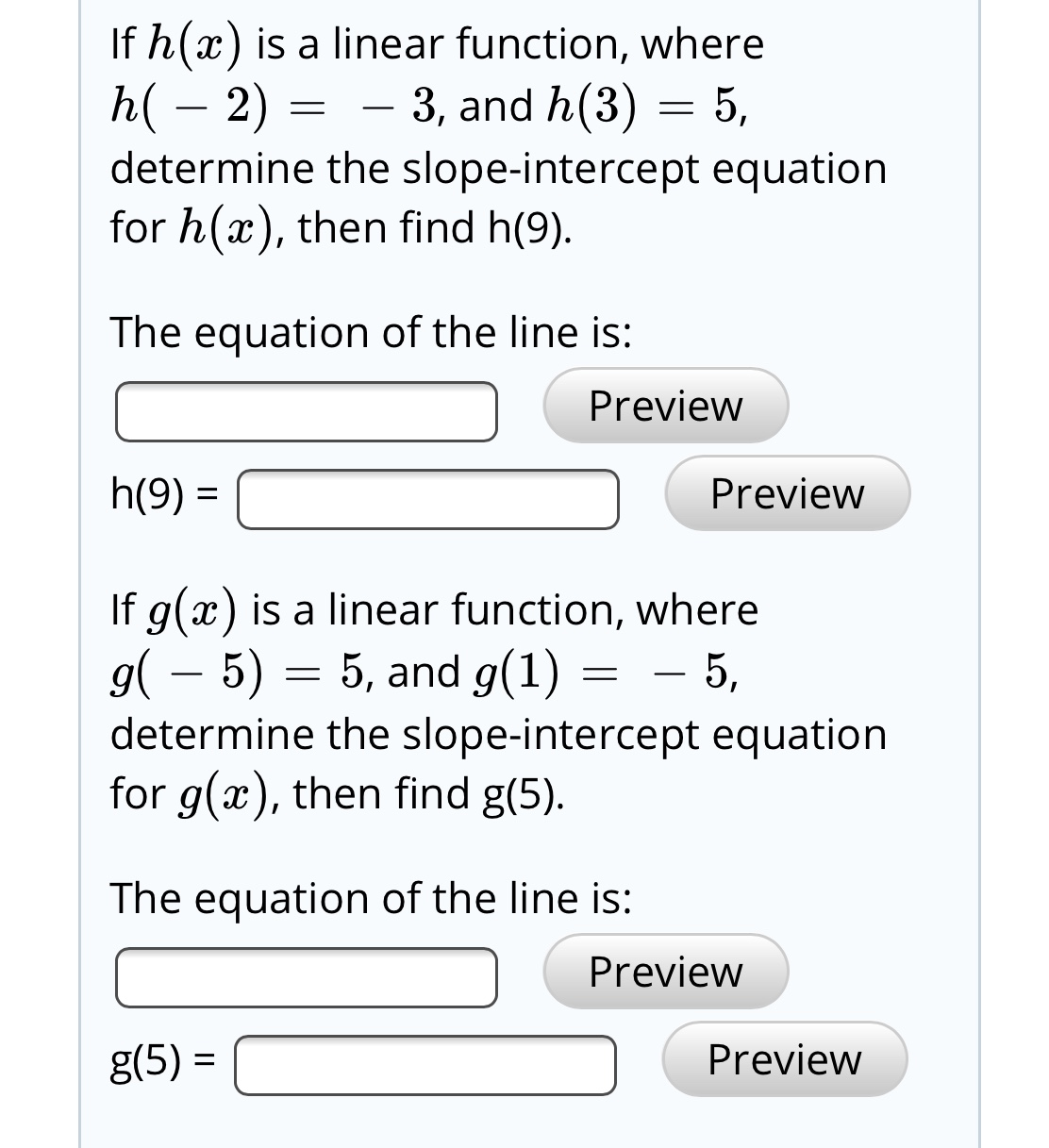# If h(x) is a linear function, whereh( – 2) =determine the slope-intercept equationfor h(x), then find h(9).3, and h(3) = 5,The equation of the line is:Previewh(9) =PreviewIf g(x) is a linear function, whereg( – 5) = 5, and g(1)determine the slope-intercept equationfor g(x), then find g(5).- 5,The equation of the line is:PreviewPreviewg(5) =

Question
8 viewshelp_outlineImage TranscriptioncloseIf h(x) is a linear function, where h( – 2) = determine the slope-intercept equation for h(x), then find h(9). 3, and h(3) = 5, The equation of the line is: Preview h(9) = Preview If g(x) is a linear function, where g( – 5) = 5, and g(1) determine the slope-intercept equation for g(x), then find g(5). - 5, The equation of the line is: Preview Preview g(5) = fullscreen
check_circle

Step 1

Given that h(x) is a linear function.

Step 2

Further:

Step 3

Now for g(...

### Want to see the full answer?

See Solution

#### Want to see this answer and more?

Solutions are written by subject experts who are available 24/7. Questions are typically answered within 1 hour.*

See Solution
*Response times may vary by subject and question.
Tagged in

### Equations and In-equations